# Interest Calculation

Interest is a legitimate return on money invested and chargeable in the business world on loans and also on delayed payments. Interest can be calculated on the basis of Simple or Compound Interest. With Tally.ERP 9 you can obtain reports on interest calculated based on the configurations made. Interest is calculated on outstanding receivables or payables.

## Enable interest calculation

1. Click F11 > F1(Accounting Features) .

2. Set the option Activate interest calculation to Yes .

3. Press Ctrl+A to save the Company Operations Alteration .

## Calculate interest in simple mode

Interest calculation on outstanding balances is allowed for any ledger account. Configure the interest rate and style of calculation in the ledger master to start calculating interest on outstanding amounts.  Interest on outstanding balances can also be calculated transaction-by-transaction .

1. Go to Gateway of Tally > Accounts Info. > Ledgers > Alter (Single) > XY Bank (bank account)

2. Set Activate interest calculation? to Yes . The Interest Parameters screen appears.

3. In the Calculate Interest Based On? field select the Date Type , as required.

4. Set Include transaction date for interest calculation . This can be enabled For Amounts added and/ or For Amounts Deducted .

5. Enter the Rate of interest.

6. Select the Interest Style , as required. The rate of interest will be calculated on the basis of the interest style applied.

Note: If the interest style selected is 365-day Year , then the interest will be calculated for 365 days irrespective of the number of days in the calendar year. If Calendar Year is selected as the interest style, then the rate of interest will be calculated on 365 days or 366 days, depending on the number of days as per the calendar.

7. Select required details in other fields of Ledger Creation screen.

8. Press Ctrl+A to save.

## Calculate interest transaction-by-transaction

Interest calculation can be done bill-by-bill for accounts grouped under Sundry Debtors and Sundry Creditors .

1. In the party ledger alteration screen, set Activate interest calculation? to Yes . The Interest Parameters screen appears.

2. Set Calculate Interest Transaction-by-transaction? to Yes .

3. Set Override Parameters for each Transaction? to Yes to change the parameters during voucher or invoice entry.

4. Enter the rate of interest in the field Rate .

5. Select the Interest Style , as required. The rate of interest will be calculated on the basis of the interest style applied.

## Calculate interest in advanced mode

The advanced parameters are used when interest rates change periodically. Number of days, balance type, and applicable from date to be considered for interest calculation can be configured using advanced parameters.

1. Go to Gateway of Tally > F11: Features > Accounting Features

2. Set Activate interest calculation? to Yes .

3. Set Use advanced parameters? to Yes .

4. Press Ctrl+A to accept the changes.

Set advanced configuration for a party

1. In the party ledger master set Calculate Interest Transaction-by-Transaction? to Yes to calculate interest for each transaction.

2. Set Override Parameters for each Transaction? to Yes t o display the Interest Parameters screen while recording the transaction. The defined parameters can be modified during voucher entry.

3. Set Override advanced parameters? to Yes.

4. Enter the Rate of interest.

5. Select the Interest Style , as required. The rate of interest will be calculated on the basis of the interest style applied.

6. Select the balance on which the interest has to be calculated. Interest can be calculated on credit balance, debit balance or both credit and debit balances.

7. Select the Applicability of the interest as required. If Past Due Date is selected, then the number of days after which interest has to be applied can be specified.

8. Select the date from which interest is applicable in the Calculate From field.

9. Select the rounding method applicable in the Rounding field. Select Not Applicable for saving values as is without rounding off.

10. Define multiple slabs with different rates and applicability.

The Interest Parameters screen with multiple slabs appears as shown below: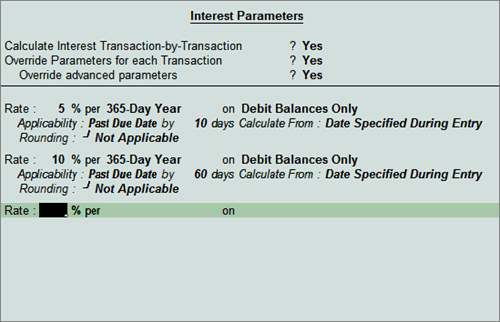11. Press Ctrl+A to accept the configuration.

12. Press Ctrl+A to save the ledger.

In case of the above configuration, interest will be calculated at 5% starting from 10th day after due date and at 10% starting from 60th day after due date. Similarly, multiple slabs can be configured with different interest style, applicability, calculate from and rounding.

## Calculate interest on Group

The Group Interest Calculation statement is a list of all accounts and sub-groups belonging to the selected group that displays the current balance in each account or sub-group and the total interest.

1. Go to Gateway of Tally> Display > Statements of Accounts > Interest Calculations > Group

2. Select a Group , E.g. Loans (Liability)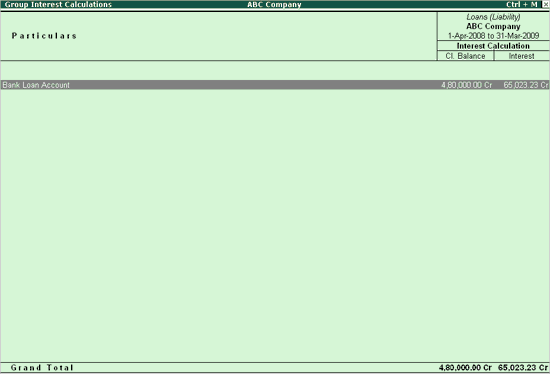Note: The Cr in the interest amount indicates that it is a liability.

3. Press Enter to drill down to the Ledger level Interest report.

## Calculate interest on Ledger Balances

You can get a report on the Ledger balances for the interest calculated.

1. Go to Gateway of Tally > Display > Statements of Accounts > Interest Calculations > Ledger

2. Select an Account, for example, Bank Loan Account . The Ledger Interest report appears as shown below:## View interest amount on invoices

You can calculate interest on each invoice for the period it is outstanding, wholly or partly. Transaction by transaction or bill-by-bill interest calculation is permitted for party accounts like accounts under the groups sundry debtors and sundry creditors. For interest calculation on each bill, Bill-wise details should be enabled for the company and the party.

The Ledger Interest report displays the interest calculated for the ledger selected. If a part of the amount is settled, then interest is calculated only on the amount pending to be repaid.

1. Go to Gateway of Tally > Display > Statements of Accounts > Interest Calculations > Ledger .

2. Select the required ledger. The Ledger Interest report appears as shown below: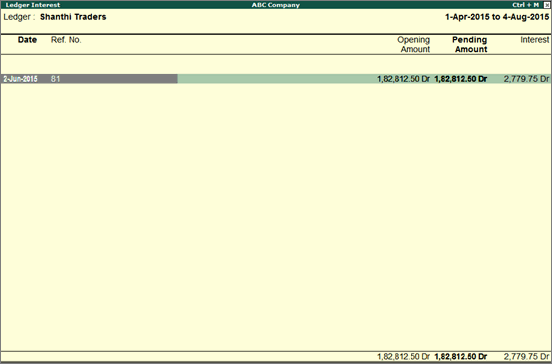3. Click F1 : Detailed to view the calculation and period.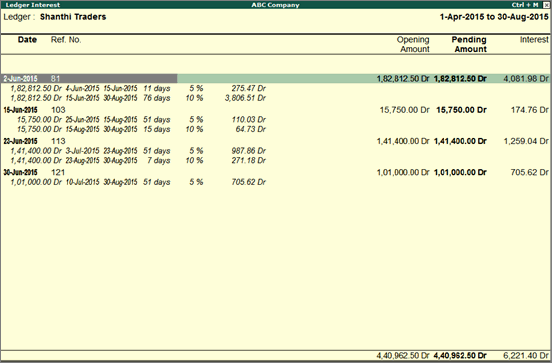Note: In case if part of the amount is settled, the interest is calculated on the remaining balance.

Now check the interest amount due.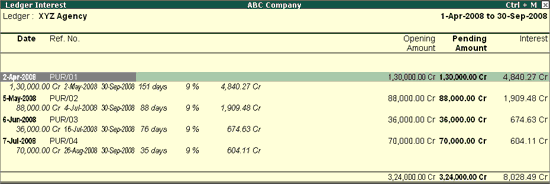Note: The Cr in the interest amount indicates that it is a liability.

The interest liability and expense can be accounted by recording a journal entry. Interest can also be recorded using debit notes and credit notes with voucher class.